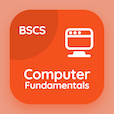Engineering Online Courses

Electromagnetic Theory Quizzes

Electromagnetic Theory Quiz PDF - Complete

# Drude Model Quiz MCQ Online p. 54

Practice Drude Model quiz questions and answers PDF, drude model trivia questions 54 to learn online Electromagnetic Theory course for online classes. Metamaterials MCQ questions, Drude Model Multiple Choice Questions (MCQ) for online college degrees. "Drude Model Quiz" PDF eBook: photon energy, chiral metamaterials, boundary conditions, dielectric permittivity, drude model test prep for grad school interview questions.

"Drude model is an application of" MCQ PDF: potential theory, kinetic theory, force theory, and power theory for undergraduate engineering schools. Solve metamaterials questions and answers to improve problem solving skills for online high school college acceptance.

## Trivia Quiz on Drude Model MCQs

MCQ: Drude model is an application of

Kinetic theory
Potential theory
Force theory
Power theory

MCQ: Relative permitivity '∊sr' in dielectric is also known as

relative constant
static constant
dynamic constant
dielectric constant

MCQ: Weighted combination of Dirichlet boundary conditions and Neumann boundary conditions are

Combined boundary condition
Robin boundary condition
Cauchy boundary condition
Mixed boundary condition

MCQ: Swiss roll structure of chiral metamaterial has resonance due to

capacitance
resistance
inductance
reductance

MCQ: The equation for photon energy 'E' is

hc/⋋
h⋋/c
c⋋/h
ch⋋

### More Quizzes from Electromagnetic Theory Course